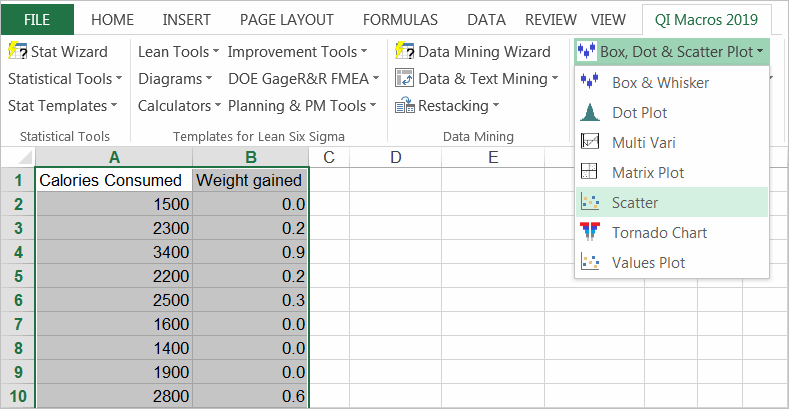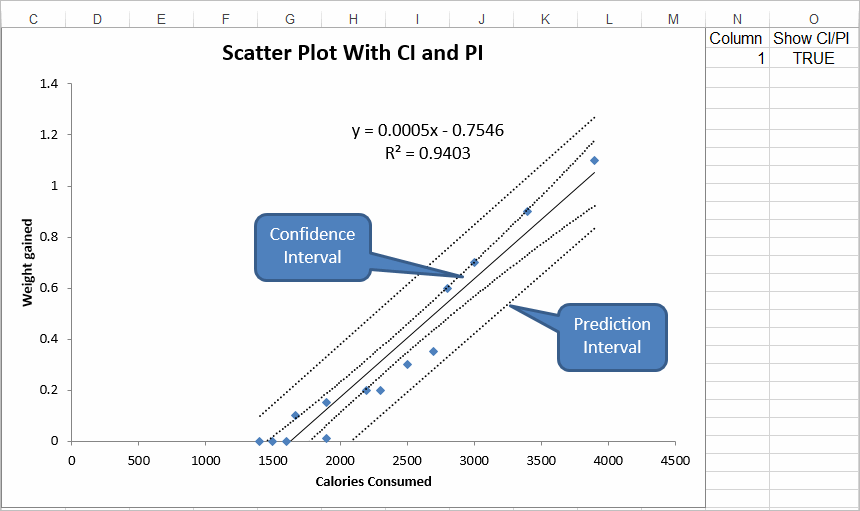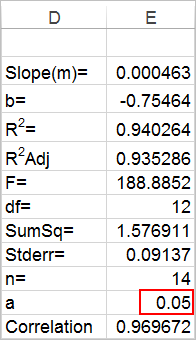# Need a Scatter Plot with Confidence and Prediction Intervals?

## QI Macros draws scatter diagrams with both intervals in seconds

### Go Deeper:

• Confidence Intervals: Provide a view into the uncertainty when estimating the mean.
• Prediction Intervals: Account for variation in the Y values around the mean.

### Step by step example of drawing a scatter plot in QI Macros

1. Select your data, then select Scatter Plot from QI Macros menu.2. QI Macros will perform all of the calculations and create a scatter plot with a trend line and lines for the confidence interval and prediction interval. QI Macros scatter plot also displays calculations for the correlation of determination.

If R 2 is greater than .80, you have a strong correlation.3. Once the scatter diagram is drawn, you can:
• Swap the order of the X axis and Y axis
• Show/Hide the CI/PI lines using the True/False option on the worksheet.• Change the alpha value to adjust the confidence intervals (0.05 = 95% confidence, 0.01 = 99%).
Just move the chart to reveal the calculations behind it:
•